# Long Division

Below is the process written out in full.
You will often see other versions, which are generally just a shortened version of the process below
.
You can also see this done in Long Division Animation.

Let's see how it is done with:• the number to be divided into is called the dividend
• The number which divides the other number is called the divisor

And here we go: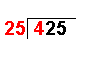4 ÷ 25 = 0 remainder 4 The first digit of the dividend (4) is divided by the divisor.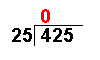The whole number result is placed at the top. Any remainders are ignored at this point.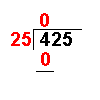25 × 0 = 0 The answer from the first operation is multiplied by the divisor. The result is placed under the number divided into.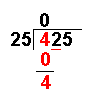4 − 0 = 4 Now we subtract the bottom number from the top number.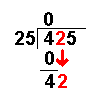Bring down the next digit of the dividend.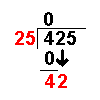42 ÷ 25 = 1 remainder 17 Divide this number by the divisor.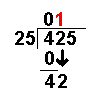The whole number result is placed at the top. Any remainders are ignored at this point.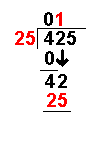25 × 1 = 25 The answer from the above operation is multiplied by the divisor. The result is placed under the last number divided into.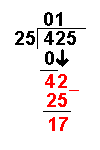42 − 25 = 17 Now we subtract the bottom number from the top number.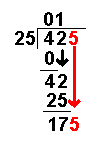Bring down the next digit of the dividend.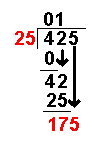175 ÷ 25 = 7 remainder 0 Divide this number by the divisor.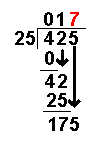The whole number result is placed at the top. Any remainders are ignored at this point.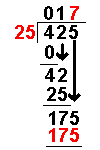25 × 7 = 175 The answer from the above operation is multiplied by the divisor. The result is placed under the number divided into.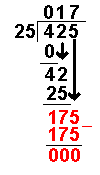175 − 175 = 0 Now we subtract the bottom number from the top number. There are no more digits to bring down. The answer must be 17

Long Division Worksheets